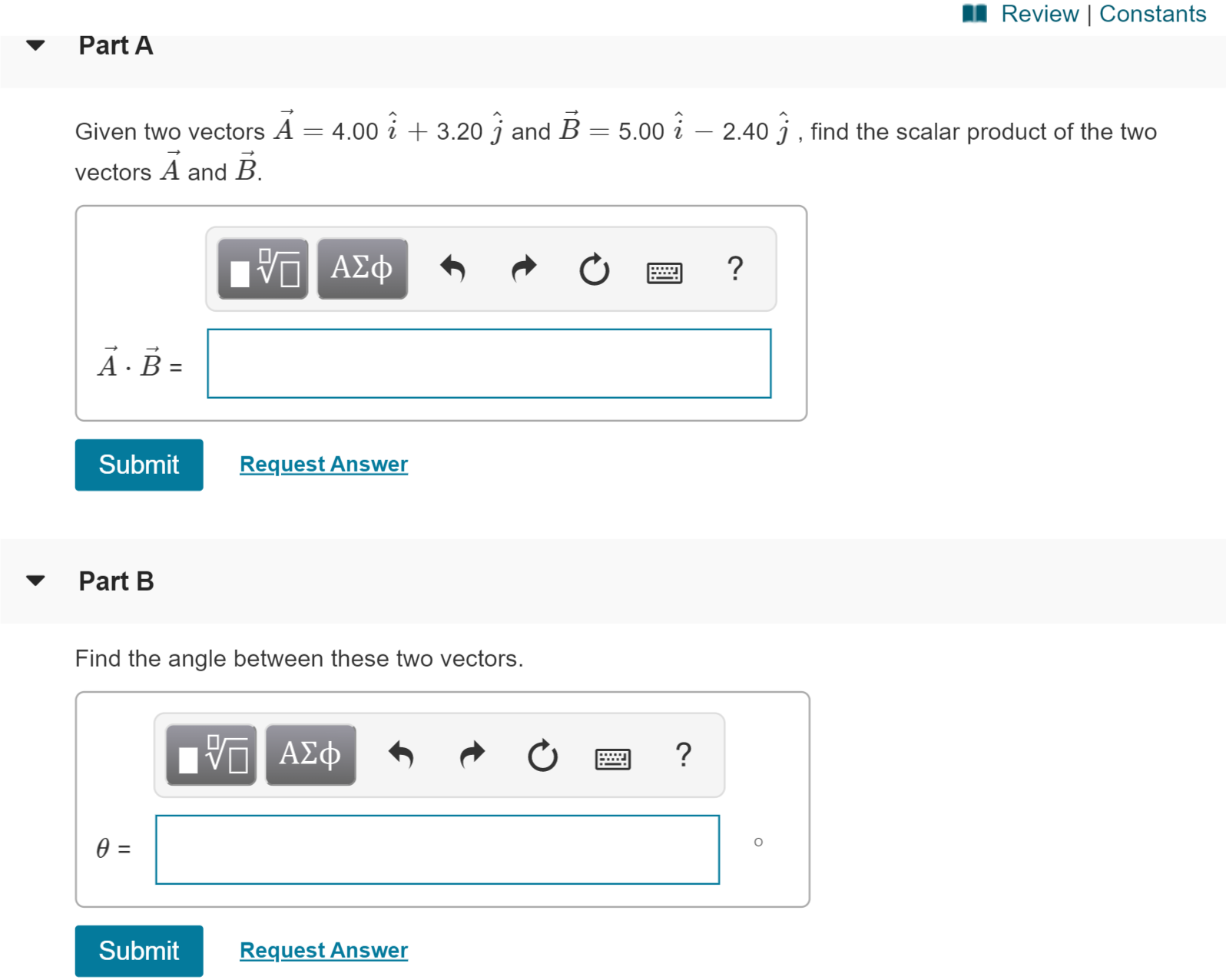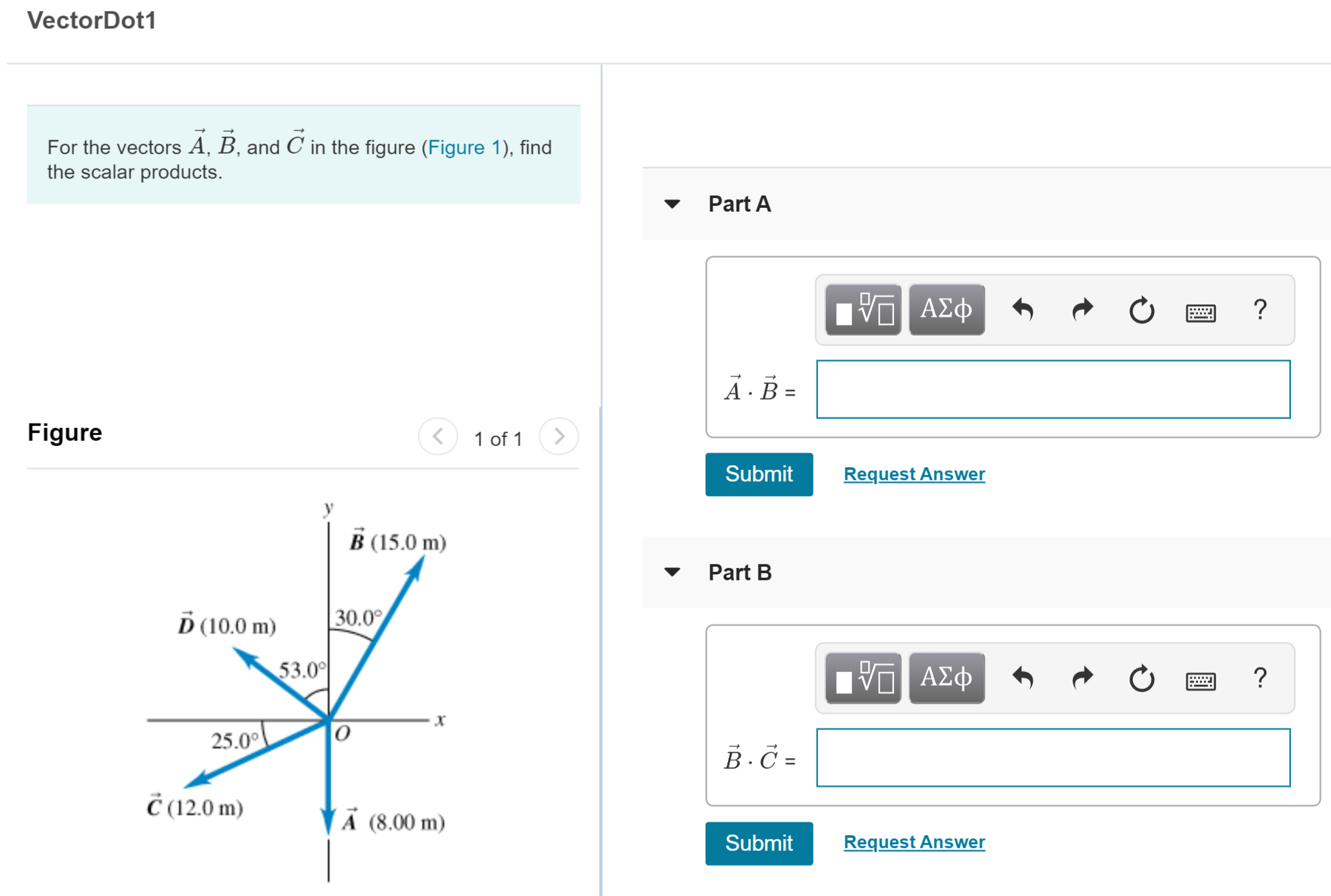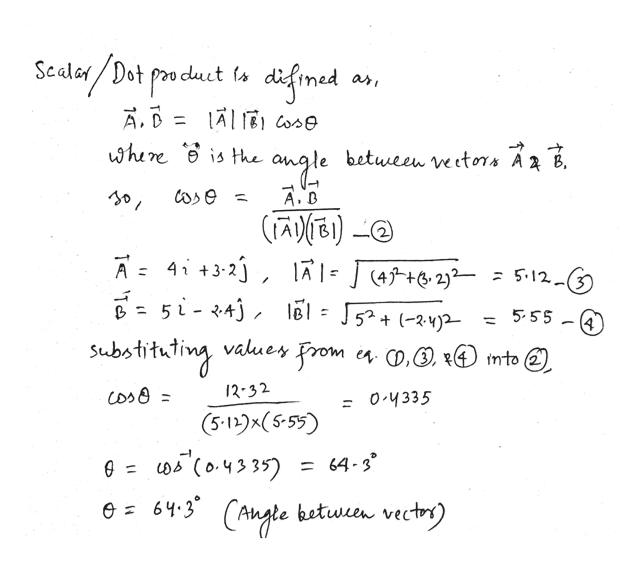# Review | ConstantsPart AGiven two vectors A = 4.00 i 3.20 j and B = 5.00 i - 2.40 j , find the scalar product of the twovectors A and BΑΣφA.B=Request AnswerSubmitPart BFind the angle between these two vectors.Α φ0 =SubmitRequest Answer VectorDot1For the vectors A, B, and C in the figure (Figure 1), findthe scalar products.Part AΑΣφFigure>1 of 1Request AnswerSubmitB (15.0m)Part B30.0D (10.0 m)53.00ΑΣφ?25.0В.С-Č(12.0 m)VA (8.00 m)SubmitRequest Answer

Question
58 viewshelp_outlineImage TranscriptioncloseReview | Constants Part A Given two vectors A = 4.00 i 3.20 j and B = 5.00 i - 2.40 j , find the scalar product of the two vectors A and B ΑΣφ A.B= Request Answer Submit Part B Find the angle between these two vectors. Α φ 0 = Submit Request Answer fullscreenhelp_outlineImage TranscriptioncloseVectorDot1 For the vectors A, B, and C in the figure (Figure 1), find the scalar products. Part A ΑΣφ Figure > 1 of 1 Request Answer Submit B (15.0m) Part B 30.0 D (10.0 m) 53.00 ΑΣφ ? 25.0 В.С- Č(12.0 m) VA (8.00 m) Submit Request Answer fullscreen
check_circle

star
star
star
star
star
1 Rating
Step 1

Given:

Vector A = 4 i + 3.2 j

Vector B = 5 i – 2.4 j

Step 2

(a) Calculating the scalar product of given vectors:

Step 3

(b) Calculating the angle b...help_outlineImage TranscriptioncloseScalar/Dat poa duct ta afmed iofmed A. D A Ei Cose where is Hhe angle betuween ve etors A 2 b. ari A.B (A)(E) 4i +3-2 A ] 4t+&2}2 5- = 1 abotithling A = 512-(3 52+2)2 = 555 4 values From ea 0, x into 2) 12-32 os 0 4335 (5-12)(5-55) ) (০-५११5) = 64-3 64.3 (Angle between vectry) fullscreen

### Want to see the full answer?

See Solution

#### Want to see this answer and more?

Solutions are written by subject experts who are available 24/7. Questions are typically answered within 1 hour.*

See Solution
*Response times may vary by subject and question.
Tagged in

### Physics